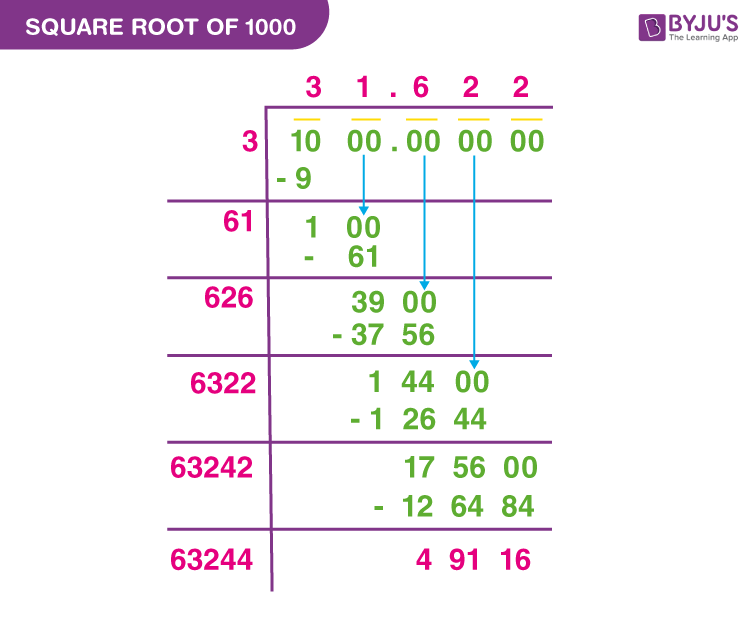# Square Root of 1000

The square root of 1000 is a number, which when multiplied by itself results in the original number 1000. The value of the square root of 1000 is an irrational number, as it cannot be expressed in the form of p/q. In this article, we are going to discuss the value of the square root of 1000 in decimal form, radical form and the methods such as prime factorization method and long division to find the square root of 1000 in detail.

## What is the Square Root of 1000?

If a number is multiplied by itself and gives the result as 1000, then the number is the square root 1000. The square root of 1000 is symbolically expressed as √1000.

Hence, √1000 = √(Number × Number)

Thus, if we multiply the number 31.622 two times, we get the original value 1000.

(i.e) √1000 = √(31.622× 31.622)

√1000 = √(31.622)2

Now, remove square and square root, we get

√1000 = ± 31.622

Square Root of 1000 in Decimal Form: 31.622.

## Square Root of 1000 in Radical Form

To express the square root of 1000 in the simplest radical form, write the prime factorization of 1000. The prime factorization of 1000 is 2 × 2 × 2 × 5 × 5 × 5. Thus, the simplest radical form of the square root of 1000 is 10√10.

Square Root of 1000 in Radical Form: 10√10.

## Square Root of 1000 by Prime Factorization Method

To find the square root of 1000 using the prime factorization method, we need to know the prime factorization of 1000. Thus, the prime factorization of 1000 is 2 × 2 × 2 × 5 × 5 × 5.

Thus, √1000 = √(2 × 2 × 2 × 5 × 5 × 5)

√1000 = (√2).(√5).(√2)2.(√5)2

√1000 = √2. √5 (2×5)

√1000 = 10.√2. √5

We know that,

√2 = 1.414

√5 = 2.236

Now, substitute the values of √2 and √5 in the above equation.

√1000 = 10(1.414)(2.236)

√1000 = 31. 622(approximately)

Hence, the square root of 1000 in decimal form is approximately equal to 31.622.

## Square Root of 1000 by Long Division Method

The procedure to find the square root of 1000 using the long division method is given as follows:

Step 1: Write the number 1000 in decimal form. To find the exact value of the square root of 1000, add 6 zeros after the decimal point. Hence, 1000 in decimal form is 1000.000000. Now, pair the number 1000 from right to left by putting the bar on the top of the number.

Step 2: Now, divide the number 10 by a number, such that the product of the same number should be less than or equal to 10. Thus, 3×3=9, which is less than 10. Thus, we obtained the quotient = 3 and remainder = 1.

Step 3: Double the quotient value, so we get 6 and assume that 60 is the new divisor. Now, bring down the value 00 for division operation. So, the new dividend obtained is 100. Now, find the number, such that (60 + new number) × new number should give the product value, that should be less than or equal to 100. Hence, (60+1) × 1 = 61, which is less than 100.

Step 4: Now subtract 61 from 100, and we get 39 as the new reminder, and 31 as a quotient.

Step 5: The new quotient obtained is 31, and double that. Hence, we get 62 and assume that 620 is our new divisor. Now, bring down the two zeros and, we have 3900 as the new dividend.

Step 6: Find the number, such that (620 + new number) × new number should give the product value, that should be less than or equal to 3900. Thus, (620+6)× 6 = 3756, which is less than 3900.

Step 7: Subtract 3756 from 3900, and we get 144 as the new reminder.

Step 8: Continue this process until we get the approximate value of the square root of 1000 up to three decimal places. (Note: keep the decimal point in the quotient value after bringing down all the values in the dividend).

Step 9: Thus, the approximate value of the square root of 1000, √1000 is 31.622.## Video Lessons on Square Roots

### Visualising square roots### Finding Square roots### Examples

Example 1:

Simplify [10√10 ×√1000]+150.

Solution:

Given: [10√10 ×√1000]+150.

We know that the square root of 1000 in radical form is 10√10.

Now, substitute the value in the expression, we get:

[10√10 ×√1000]+150 = [10√10 ×10√10]+150 [10√10 ×√1000]+150 = 1000+150 [10√10 ×√1000]+150 = 1150.

Example 2:

Find the value of k, if 75k+√1000 – 20 = 2130.

Solution:

Given: 75k+√1000 – 20 = 2130

We know that √1000 = 31.622.

Now, substitute the value in the given equation, we get

75k+31.622 – 20 = 2130

75k+11.622 = 2130

75k = 2130 – 11.622

75k = 2118.378

k = 2118.378/75

k = 28.24504

## Frequently Asked Questions on Square Root of 1000

Q1

### What is the value of the square root of 1000?

The value of the square root of 1000 is approximately equal to 31.622.

Q2

### What is the square root of 1000 in radical form?

The square root of 1000 in radical form is 10√10.

Q3

### Is 1000 a perfect cube?

Yes, 1000 is a perfect cube, as it can be expressed in the form of the product of three equal integers. (i.e) 1000 = 10×10×10.

Q4

### What is the value of the square of square root of 1000?

The square of square root of 1000 is 1000.

(i.e) (√1000)2 = 1000 (On removing square and square root, we get 1000).

Q5

### What is √10 plus square root of 1000?

We know that the square root of 1000 in radical form is 10√10.
Hence, √10+10√10 = 11√10.

Test your Knowledge on Square Root Of 1000